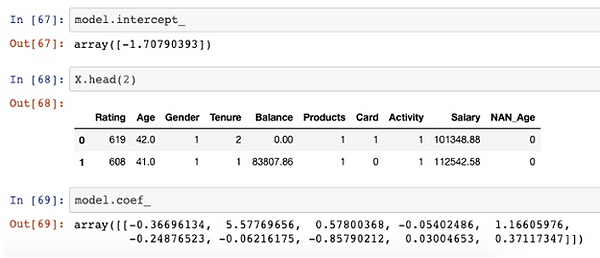top of page

Soon, Einstein Discovery will be available to do Machine Learning directly in Tableau. Until then, we have to work with different solutions.

###### How to add a Multiple-Linear Regression in Tableau

In Tableau, adding a simple linear regression (a.k.a., trend) with two variables is easy to implement and visualize. Adding a multiple linear regression with more than two variables required a bit of help from R or Python. In my example below, we extract the intercept and coefficients from R, implement them in a Calculated Field, and link them with Parameters.Of course, visualizing a multiple linear regression is almost impossible. However, we can use multiple linear regression to achieve advanced calculations such as expected returns from an advertising campaign or dynamic pricing. Some call this prediction. However, unlike forecasting, with multiple linear regression, we should only interpolate and not extrapolate. In other words, with multiple linear regression, we can only stay within the range in which we trained our algorithm.

###### Industry 4.0 - Predictive Maintenance in Tableau

One serious challenge for businesses is implementing Machine Learning in production. Tableau as a platform is almost unrivaled in giving access to data in production. For example, once in production, users can access dashboards from desktops, tablets, and even mobile phones. Dashboards can be easily modified for specific needs including data-driven email alerts.

Unfortunately, implementing a classification algorithm (Logistic Regression, Decision Tree, Random Forest ...) is not straightforward. Below is an image from an Industry 4.0 algorithm implemented in Tableau using Python in Google Colab. "Risk" refers to the probability of machine failure.###### Machine Learning directly in Tableau via R or Python

To implement a Machine Learning algorithm (e.g., Logistic Regression) in Tableau, one possible technique is to use TabPy, an external API. Many companies don't allow to the installation of third-party libraries on their Tableau Server. Those policies are understandable. One way is to "convert" the prediction directly into Tableau's Calculated Field.

For example, the following Calculated Field predicts the probability, whether a customer churns (i.e., leaves us as a customer)Similar to linear regression, the coefficients from a logistic regression can be extracted and converted into a Calculated Field. Below is an example from the coefficient and the coefficients from logistic regression (Python).The huge advantage of this technique? No need for any external code such as TabPy. Additionally, using a machine learning algorithm in production is not that simple. One nasty challenge is that you have to normalize new data based on the training and testing. With Python, one solution is to pickle.

With this proposed technique, we "only" need to normalize the data directly in Tableau. In this case, we are normalizing the data with the MinMax scaler (there are different techniques though).

Below is the MinMax scaling in Tableau which brings each measure to a range of 0 - 1:bottom of page